# Real numbers, numerical sets

There are several sets of numbers, which will be discussed below.

## The set of real numbers

Effect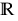.

Rational and irrational numbers form the real numbers. They are numbers that can be represented in the form of infinite decimal fractions.

## The set of Complex numbers

Effect.

It is numbers of the form, where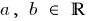;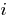is the imaginary unit.

## The set of Rational numbers

Effect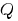.

Combining whole numbers with a decimal, get a rational number

Rational numbers can be represented in the form of irreducible fraction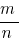, where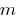integer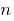is a natural number written as infinite periodic decimal fractions).

## The set of Irrational numbers

They are numbers that can be written in the form of an infinite non-periodic decimal fractions.

## The set of integers

Effect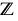.

Include natural numbers, their opposites numbers (negative) and the number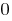.

## The set of natural numbers

Effect.

They are positive integers.

## Fractional numbers

It's a numbers composed of integer fractions of a unit.— common fractions;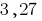— decimals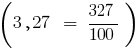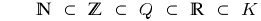Tags:
Chapter:
Versions in other languages:
Share with friends: